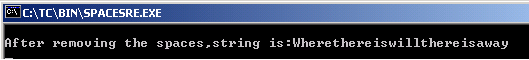Ads

Tutorials

# C String Remove Spaces

In this section, you will study how to remove spaces from the string.

In this section, you will study how to remove spaces from the string.

# C String Remove Spaces

In this section, you will study how to remove spaces from the string. You can see in the given example, we have define the string and passed it into two character pointers. The expression p1 = ch, resets the pointer to start. If any punctuation character or whitespace character is found in the *p1 till p1, to be not equal to 0, then ++p1 moves over a whole character otherwise copy the character using the expression *p2++ = *p1++;. The *p2 =

0; appends the string terminator.

ispunct()- finds the punctuation character.

isspace()-finds the whitespace character.

Here is the code:

SPACESRE.C

 ``` #include  #include  #include  void main() {   char ch = "Where there is will,there is a way.";   char *p1 = ch;   char *p2 = ch;   p1 = ch; �  while(*p1 != 0) {   if(ispunct(*p1) || isspace(*p1)) {   ++p1;   }   else   *p2++ = *p1++;�   }   *p2 = 0;�   printf("\nAfter removing the spaces,string is:%s\n", ch);   getch(); }```

Output will be displayed as:Download Source Code:

Ads

Ads